• Windows环境下的分布式并行计算平台搭建 MATLAB并行平台底层架构 MATLAB并行平台搭建 并行程序的编写与实例 实验室MATLAB并行集群 * MATLAB并行平台底层架构一 并行平台上的三种角色 警察维护整个平台 (mdce底层维护...
• 做一个趋势交易的模型训练，准备在参数空间暴力找最优解。...果然matlab没有经得住考验，跑了两个小时突然挂了。准备用并行的方法看看能不能优化性能，短时间跑结束。  先查官方的帮助文档
  做一个趋势交易的模型训练，准备在参数空间暴力找最优解。学艺不精，不太会参数空间迭代找全局最优解。果然matlab没有经得住考验，跑了两个小时突然挂了。准备用并行的方法看看能不能优化性能，短时间跑结束。
先查官方的帮助文档


展开全文• MATLAB并行运算和分布式运算的基本操作-解MATLAB并行运算和分布式运算的基本操作.pdf 这个文档给大家介绍如何在实验室搭建分布式运算平台，及其配置。第一次给大家分享资源，但还不太熟悉怎么操作，大家见谅。
• Matlab并行计算(MATLAB分布式运算引擎)和Parallel Computing Toolbox™（并行计算工具箱），充分利用计算机集群的硬件资源来加速你的MATLAB应用程序。
• matlab云计算课程-matlab并行计算讲座.pdf 魏老师讲座，可以学习一下！
• 报错运行matlab并行时得到如下报错：failed to start a parallel pool. (For information in addition to the causingerror, validate the profile 'local' in the Cluster Profile Manager.)Caused by:Error ...
报错运行matlab做并行时得到如下报错：failed to start a parallel pool. (For information in addition to the causingerror, validate the profile 'local' in the Cluster Profile Manager.)Caused by:Error using parallel.internal.pool.InteractiveClient/start (line 330)Failed to start pool.Error using parallel.Cluster/createCommunicatingJob (line 82)The property "NumWorkersRange" cannot be set after submission.处理Parallel -> Manage Cluster Profile->validation results ->validate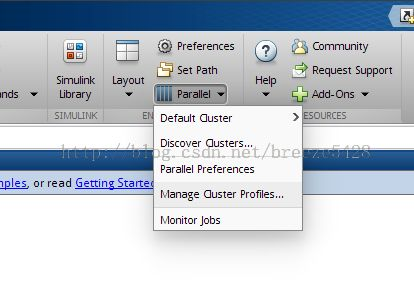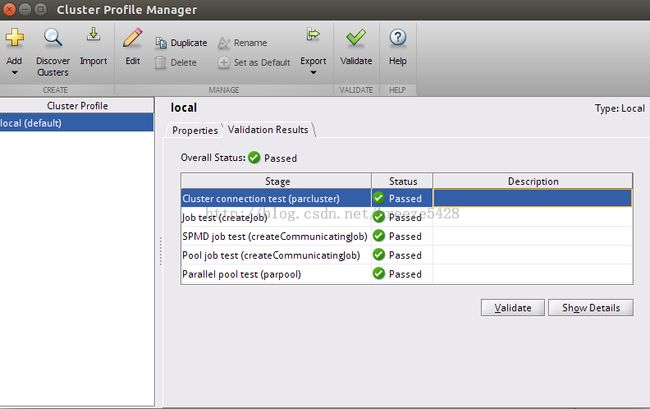权限问题http://www.matlabsky.com/forum.php?mod=viewthread&action=printable&tid=38926
展开全文• Matlab并行工具箱学习总结，很好的matlab并行学习总结，对用到matlab并行操作的用户很有帮助
• ## Matlab并行编程

千次阅读 2017-04-27 19:57:33
本文讲的Matlab并行编程包括并行for循环和数据并行（同一个程序运行在不同的数据上），分别对应于parfor、spmd方法 1. 并行方法 parpool %开启并行池（parallel pool） %parallel program delete(gcp('nocreate')...
本文讲的Matlab并行编程包括并行for循环和数据并行（同一个程序运行在不同的数据上），分别对应于parfor、spmd方法
1. 并行方法

parpool  %开启并行池（parallel pool）
%parallel program
delete(gcp('nocreate'))  % 关闭并行池
上面就是Matlab并行编程的形式。你的计算机有几个核，parpool就会开启几个worker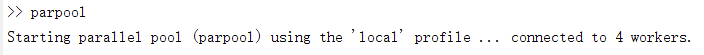当然，你也可以设定worker的数目，如这样，开启2个worker

parpool('local',2)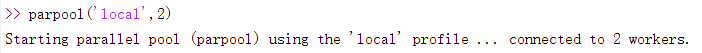至于中间的并行程序就要由parfor和spmd完成。
PS：Matlab之前的并行方法 matlabpool local 2 已经过时，现在新版的Matlab要用parpool

2. parfor
parfor(parallel for-loop)用于将for循环并行处理，当你的for循环每次迭代计算量很大，并且每次迭代都是独立的话，就可以用parpool来加速，下面是一个例子：
普通for循环

tic
n = 200;
A = 500;
a = zeros(n);
for i = 1:n
a(i) = max(abs(eig(rand(A))));
end
toc运行时间为：时间已过 25.428548 秒。

若是改用parfor

tic
n = 200;
A = 500;
a = zeros(n);
parfor i = 1:n
a(i) = max(abs(eig(rand(A))));
end
toc运行时间为：时间已过 8.801395 秒。

注意，当for循环中每次迭代不独立，即某次迭代需要其它迭代的结果，以及嵌套的for循环都是不能改为parfor的。

更多内容见Matlab文档
parfor
Decide When to Use parfor

3. spmd

spmd(single program multiple data)使多个worker运行同一段代码(single
program)，但是不同的worker处理不同的数据(multiple data)，所以spmd适用于处理很大的数据。常见的情形如：

Programs that take a long time to execute — spmd lets several workers compute solutions simultaneously.

Programs operating on large data sets — spmd lets the data be distributed to multiple workers.

一个例子：在3个worker上分别创建一个随机矩阵

spmd (3)
R = rand(4,4);
end

返回的R是一个Composite对象，大小为1*3，R(1)即worker 1上创建的矩阵，R(2)即worker 2上创建的矩阵，依次类推。

其实每个worker都有一个下标labindex，范围从1到n（n为worker数目），通过labindex可以指定特定的worker。（这里插一句，下标为什么是labindex,而不是workerindex呢，因为Matlab运行并行程序时，每个worker叫做lab）,又一个例子：

spmd (3)
if labindex==1
R = rand(9,9);
else
R = rand(4,4);
end
end
此外，我们可以通过labindex指定不同的worker处理不同的数据：

spmd (3)
result = MyFunction(labdata)
end终于到了重点，这种形式应该是我们经常要用到的，每个worker load不同的data，运行同一段代码。稍微注意的一点是，这里的labdata是一个struct，不是mat矩阵，所以要用字段名称引用。当时在这纠结了好久。。。同样的，返回的result是一个Composite对象，result(i)为第i个worker的返回值。

更多详细信息请看Matlab文档：
Run
Single Programs on Multiple Data Sets
spmd
Composite


展开全文• ## matlab并行计算

千次阅读 2015-12-10 12:35:16
matlab并行计算
用到的库函数

matlabpool

打开并行计算池
tic , toc

计时，作为测试用
parafor

并行计算的循环方式
代码

定义一个耗时的函数如下

function A_cost( n )
pause(n)
fprintf('A_cost execute finished,cost %d senconds!!\n',n);
end


编写测试代码如下

%% init
clc,clear
close all

matlabpool('open' , 2); % open
%% general
tic;
for ii = 1:5
A_cost(ii);
end
t1 = toc;
fprintf('general iter cost %f seconds in sum\n' , t1);

%% parallel test
tic;
parfor ii = 1:5
A_cost(ii);
end
t1 = toc;
fprintf('parallel cost %f seconds in sum\n' , t1);
matlabpool('close'); % close


结果如下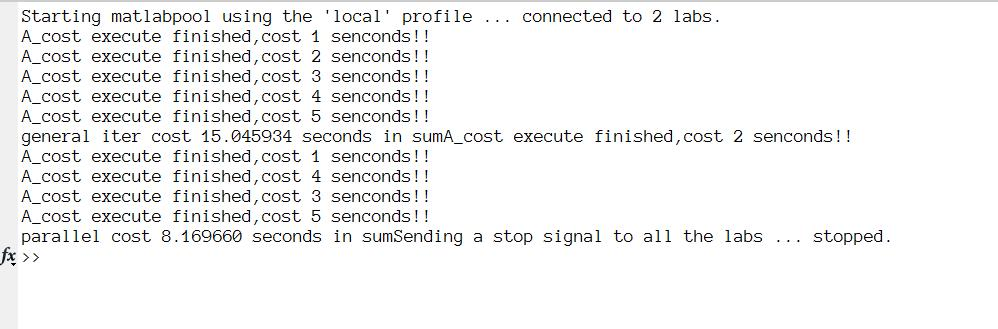注意

并行计算池打开相关

我的电脑只能打开2个并行计算池，不同的电脑应该不一样，打开的个数超过限制时，matlab会报错并提醒
循环相关

在parfor的循环过程中，不能对循环变量进行重新赋值，否则会提示错误
多次对某个值重写时，最终运行结束该值为0，如下面的程序

matlabpool('open' , 2);
tic;
parfor ii = 1:5
%ii = A_cost(ii); % will cause error
%test = ii + A_cost(ii); % test will not change
test = A_cost(ii); % test will change
end
t1 = toc;
fprintf('parallel cost %f seconds in sum\n' , t1);
matlabpool('close');

而下面的程序运行结束之后test为[1,2,3,4,5]

matlabpool('open' , 2);
tic;
parfor ii = 1:5
%ii = A_cost(ii); % will cause error
%test = ii + A_cost(ii); % test will not change
test(ii) = A_cost(ii); % test will change
end
t1 = toc;
fprintf('parallel cost %f seconds in sum\n' , t1);
matlabpool('close');


展开全文• ## Matlab并行计算学习初步

万次阅读 多人点赞 2018-08-09 08:19:57
Matlab 并行计算学习 1. 简介 高性能计算(High Performance Computing，HPC)是计算机科学的一个分支，研究并行算法和开发相关软件，致力于开发高性能计算机。可见并行计算是高性能计算的不可或缺的重要组成部分。 ...
• Matlab并行计算是指针对不同的计算平台（单机或集群），运用不同的计算工具箱。 （1）用于单机多核（最多4核）环境的并行计算工具箱（Parallel Computing Toolbox）。 （2）在集群环境中实现并行计算的分布式计算...
• matlab_集群搭建及使用.pdf MATLAB并行计算简介(是几个机子并行的).ppt MATLAB并行运算和分布式运算的基本操作-图解.pdf 家庭云服务器高阶应用：通过Matlab分布式计算....DIYPCs.pdf
• 进而实现MALTAB自动化控制多个子MATLAB运行，而且还可以利用MATLAB与MATLAB之间的消息传递方法，例如读写文本的形式传递消息，参见利用读写文本实现MATLAB应用之间的消息传递，实现多个MATLAB并行计算并相互沟通协调...
•  matlabpool或matlabpool open 打开matlab并行计算池，默认配置项为local   matlabpool open poolsize 打开matlab并行计算池的worker数为poolsize（小于配置指定的最大值），默认配置项为local  matlabpool ...
• MATLAB并行计算简介，快速完成初步入门了解！希望对大家有帮助！谢谢
• MATLAB并行计算简介(是几个机子并行的)知识讲解.ppt
• ## Matlab并行运算

千次阅读 2014-06-06 17:14:31
今天搞了一下matlab的并行计算，效果好的出乎我的意料。 本来CPU就是双核，不过以前一直注重算法，没注意并行计算的问题。今天为了在8核的dell服务器上跑程序才专门看了一下。...一、Matlab并行计算原理梗概 Matl
• MATLAB并行运算的基本操作： 并行计算-并行工具箱 parfor spmd 分布式计算-分布式计算工具箱 mdcs配置
• ## matlab并行运算方法

万次阅读 多人点赞 2017-05-31 22:47:37
matlab并行运算方法matlab在计算大数据内存以及大矩阵运算时，单核运算显然无法满足高速的运算需求。其实matlab提供多核运算的解决方案，这里先介绍最简单的两种 parfor distributed 打开并行运算的方法是parpool(4)...大数据
• MATLAB并行计算工具箱就是这种需求的产物，它能很好地实现在多核系统上进行并行运算。文章以典型的数值计算问题为例描述如何使用基本的两种并行运算方式：线程和并行for循环。典型数值计算问题为了举例说明...
• Matlab并行编程 cellfun arrayfun
• pMatlab 库文件说明，Matlab并行计算
• 刚开始接触matlab并行计算，以前旧电脑从不考虑这些的小本i7四核八线程16G内存，64位win7和matlab 2012b，任务管理器显示有8个框当用普通for循环，只有一个框接近100%，cpu使用率只有12%左右默认配置下，键入...
• 老师：matlab并行处理的时候总是报这样的错误？请问是什么原因。在做完头动矫正之后。Moving Head Motion Corrected Files:rest_closed_1122550 OKMoving Head Motion Corrected Files:rest_closed_1082398 OKMoving...
• matlab并行是给常简单地，但是有的时候使用parfor并不能开始并行。 命令行如果提示： Starting parallel pool (parpool) using the 'local' profile ... Preserving jobs with IDs: 1 because they contain crash ...
• Jeremy Kepner所写的MATLAB并行运算方法...

# matlab并行matlab 订阅